• Размер: 1.6 Mегабайта
• Количество слайдов: 11

## Описание презентации Презентация mechanical wave по слайдам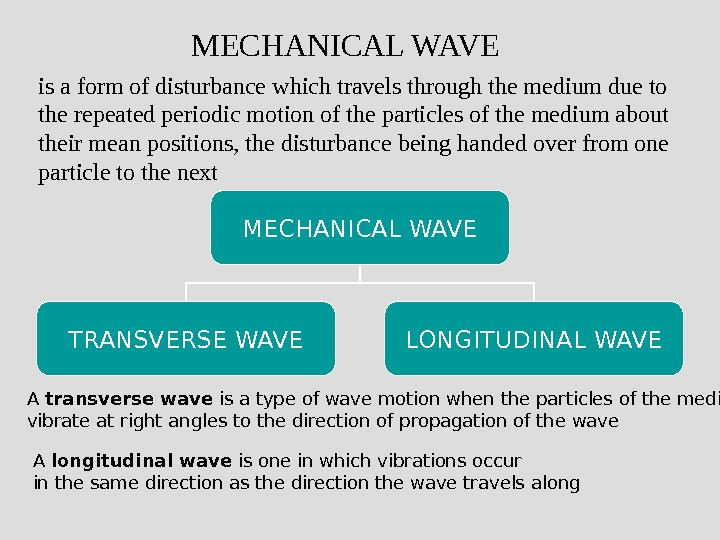is a form of disturbance which travels through the medium due to the repeated periodic motion of the particles of the medium about their mean positions, the disturbance being handed over from one particle to the next MECHANICAL WAVE TRANSVERSE WAVE LONGITUDINAL WAVE A transverse wave is a type of wave motion when the particles of the medium vibrate at right angles to the direction of propagation of the wave A longitudinal wave is one in which vibrations occur in the same direction as the direction the wave travels along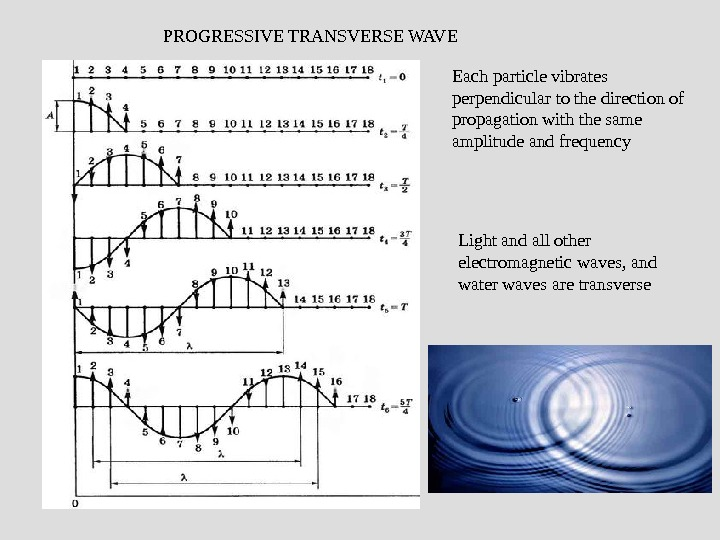PROGRESSIVE TRANSVERSE WAVE Each particle vibrates perpendicular to the direction of propagation with the same amplitude and frequency Light and all other electromagnetic waves, and water waves are transverse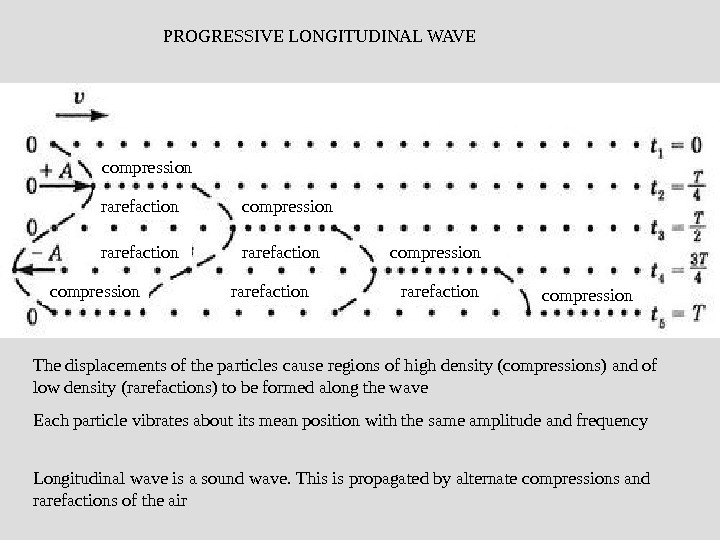: compression rarefaction compression rarefaction PROGRESSIVE LONGITUDINAL WAVE The displacements of the particles cause regions of high density (compressions) and of low density (rarefactions) to be formed along the wave Each particle vibrates about its mean position with the same amplitude and frequency Longitudinal wave is a sound wave. This is propagated by alternate compressions and rarefactions of the air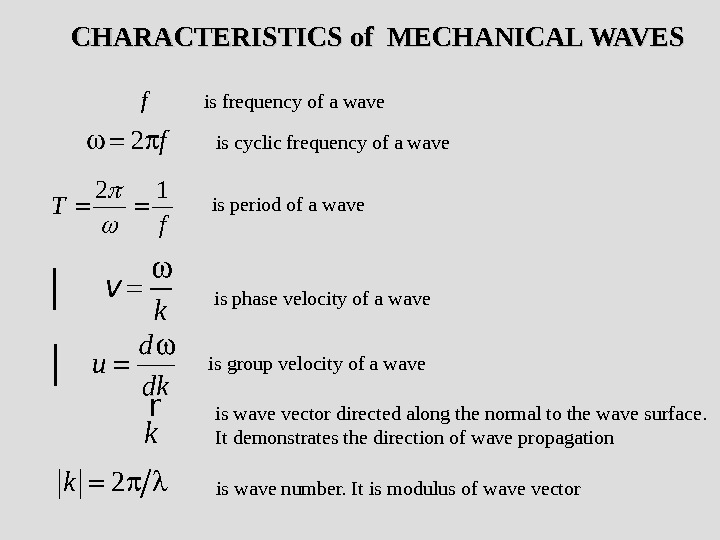CHARACTERISTICS of MECHANICAL WAVESk r f is frequency of a wave is cyclic frequency of a wave f. T 12 is period of a wave is phase velocity of a wave is group velocity of a wave is wave vector directed along the normal to the wave surface. It demonstrates the direction of wave propagation is wave number. It is modulus of wave vector 2 k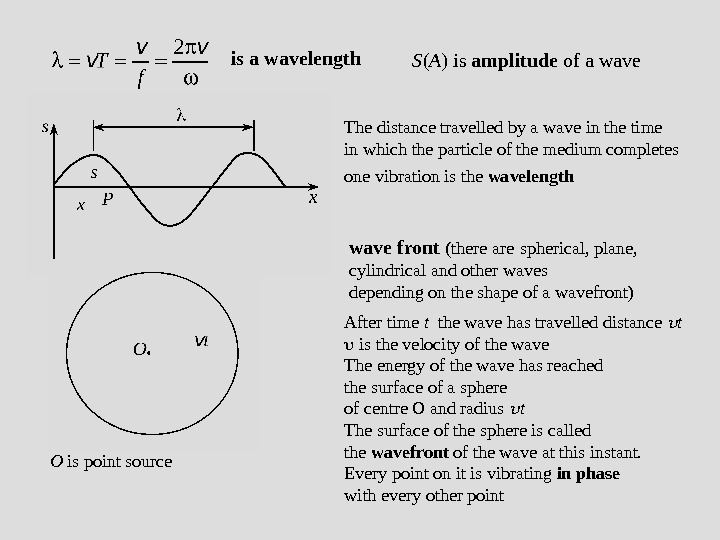is a wavelength The distance travelled by a wave in the time in which the particle of the medium completes one vibration is the wavelength O is point source After time t the wave has travelled distance t is the velocity of the wave The energy of the wave has reached the surface of a sphere of centre O and radius t The surface of the sphere is called the wavefront of the wave at this instant. Every point on it is vibrating in phase with every other point wave front ( there are spherical, plane, cylindrical and other waves depending on the shape of a wavefront) S ( A ) is amplitude of a wave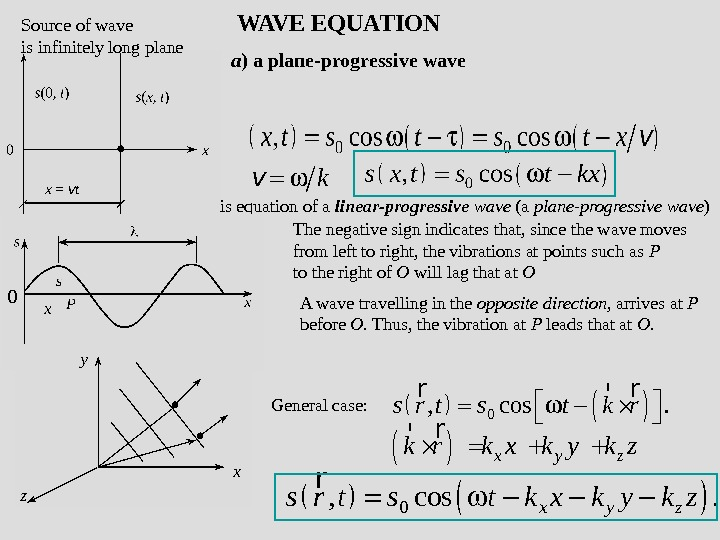WAVE EQUATION Source of wave is infinitely long plane is equation of a linear-progressive wave (a plane-progressive wave ) The negative sign indicates that, since the wave moves from left to right, the vibrations at points such as P to the right of O will lag that at O A wave travelling in the opposite direction , arrives at P before O. Thus, the vibration at P leads that at O. 00, cos. s r t s t k r rr r x y zk r k x k y k z rr 0, cos. x y zs r t s t k x k y k z r General case: a ) a plane-progressive wave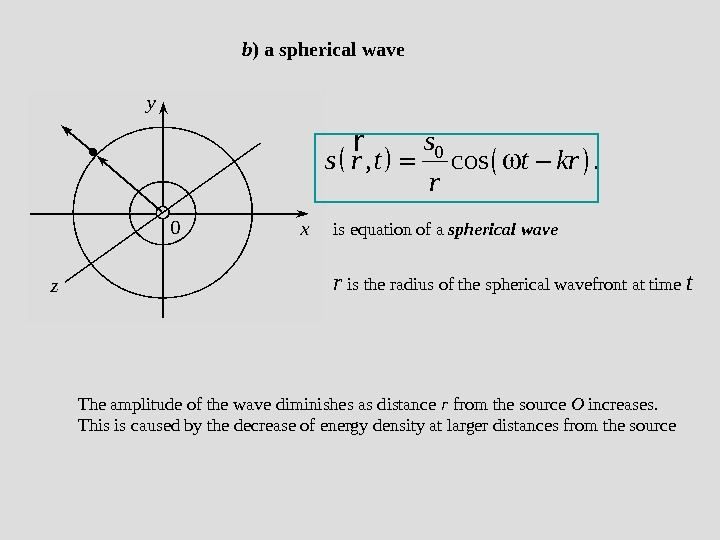b ) a spherical wave 0 , cos. s s r t t kr r r The amplitude of the wave diminishes as distance r from the source O increases. This is caused by the decrease of energy density at larger distances from the source r is the radius of the spherical wavefront at time tis equation of a spherical wave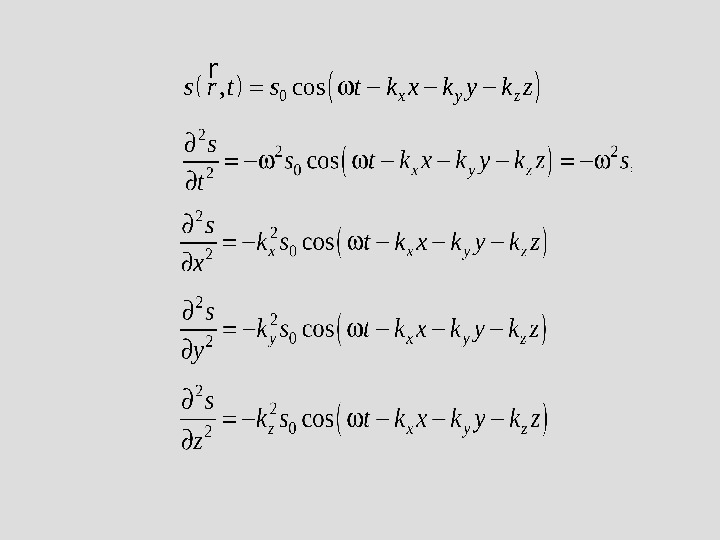0, cosx y zs r t s t k x k y k z r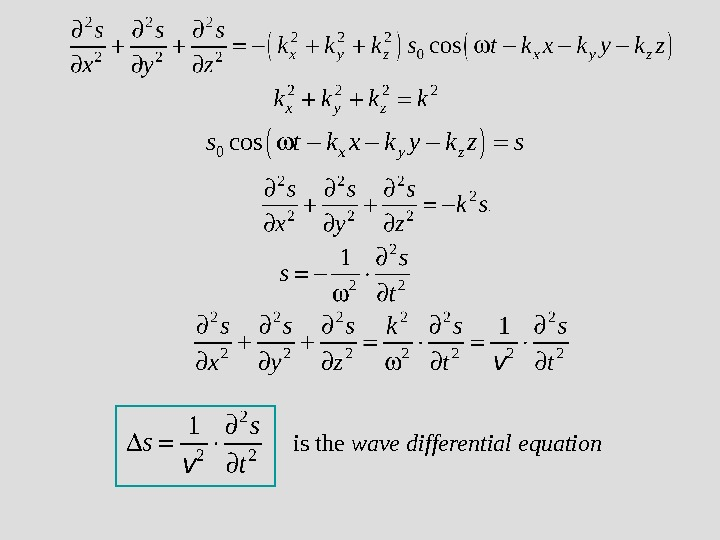is the wave differential equation 0 cosx y zs t k x k y k z s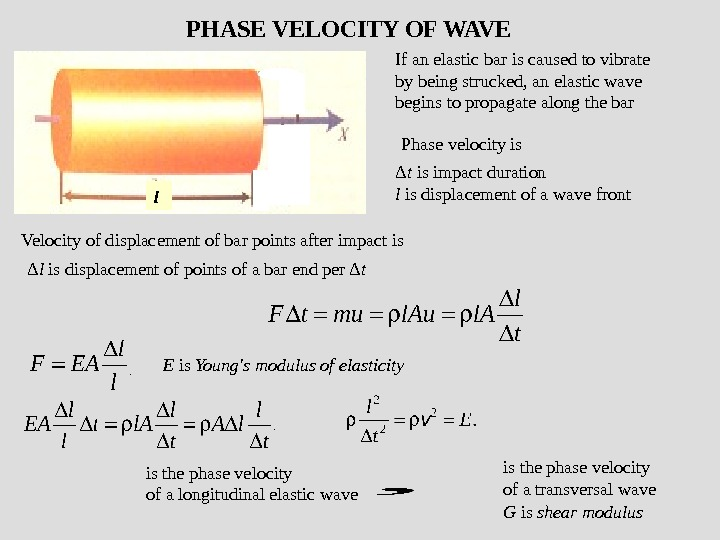If an elastic bar is caused to vibrate by being strucked, an elastic wave begins to propagate along the bar l Phase velocity is. PHASE VELOCITY OF WAVE Δ t is impact duration l is displacement of a wave front Velocity of displacement of bar points after impact is Δ l is displacement of points of a bar end per Δ t E is Young’s modulus of elasticity is the phase velocity of a longitudinal elastic wave is the phase velocity of a transversal wave G is shear modulus